# Calorimetry

May. 17, 2015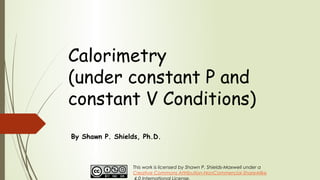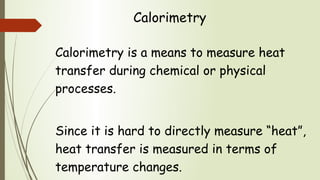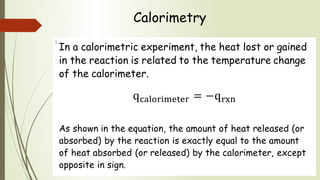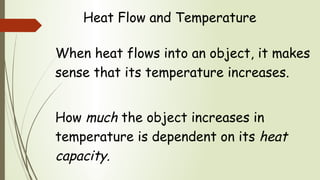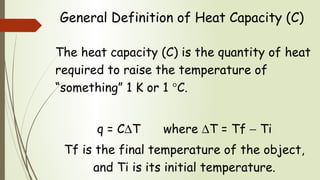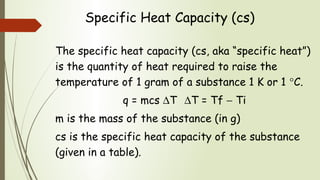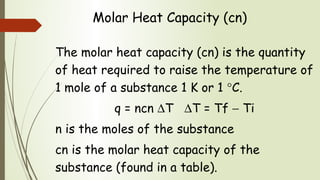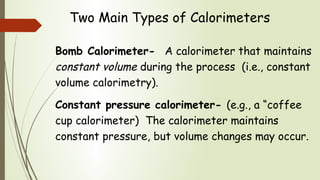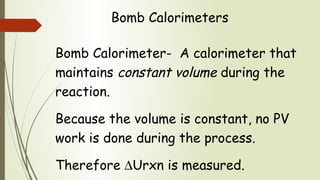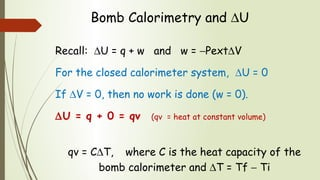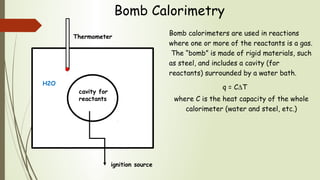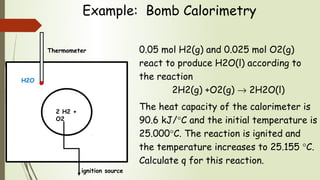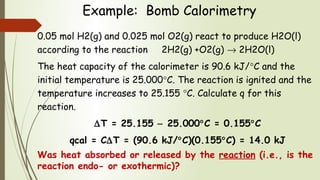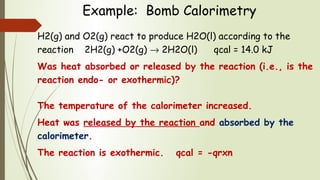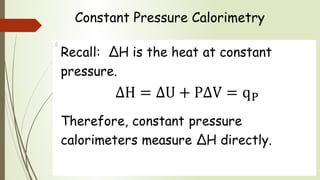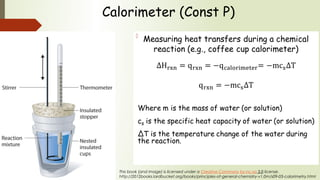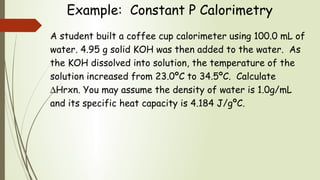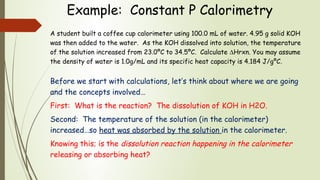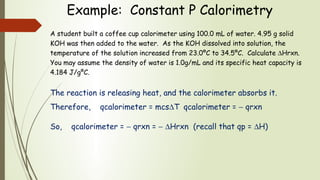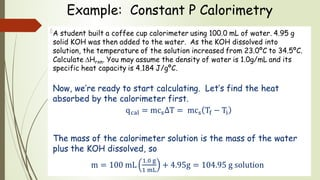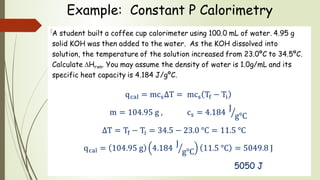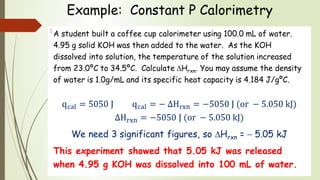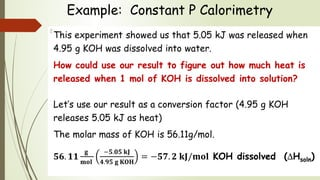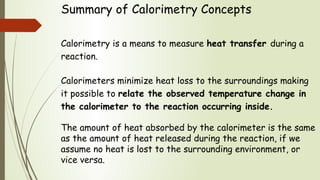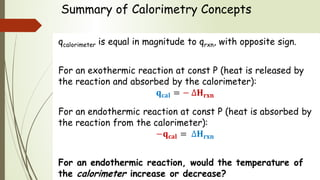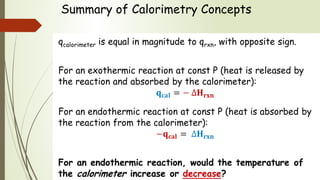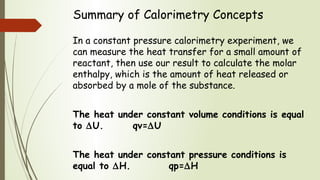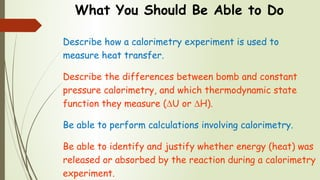1 of 28

### Calorimetry

1. Calorimetry (under constant P and constant V Conditions) By Shawn P. Shields, Ph.D. This work is licensed by Shawn P. Shields-Maxwell under a Creative Commons Attribution-NonCommercial-ShareAlike
2. Calorimetry Calorimetry is a means to measure heat transfer during chemical or physical processes. Since it is hard to directly measure “heat”, heat transfer is measured in terms of temperature changes.
3. Calorimetry In a calorimetric experiment, the heat lost or gained in the reaction is related to the temperature change of the calorimeter. As shown in the equation, the amount of heat released (or absorbed) by the reaction is exactly equal to the amount of heat absorbed (or released) by the calorimeter, except opposite in sign. 
4. Heat Flow and Temperature When heat flows into an object, it makes sense that its temperature increases. How much the object increases in temperature is dependent on its heat capacity.
5. General Definition of Heat Capacity (C) The heat capacity (C) is the quantity of heat required to raise the temperature of “something” 1 K or 1 °C. q = C∆T where ∆T = Tf − Ti Tf is the final temperature of the object, and Ti is its initial temperature.
6. Specific Heat Capacity (cs) The specific heat capacity (cs, aka “specific heat”) is the quantity of heat required to raise the temperature of 1 gram of a substance 1 K or 1 °C. q = mcs ∆T ∆T = Tf − Ti m is the mass of the substance (in g) cs is the specific heat capacity of the substance (given in a table).
7. Molar Heat Capacity (cn) The molar heat capacity (cn) is the quantity of heat required to raise the temperature of 1 mole of a substance 1 K or 1 °C. q = ncn ∆T ∆T = Tf − Ti n is the moles of the substance cn is the molar heat capacity of the substance (found in a table).
8. Two Main Types of Calorimeters Bomb Calorimeter- A calorimeter that maintains constant volume during the process (i.e., constant volume calorimetry). Constant pressure calorimeter- (e.g., a “coffee cup calorimeter) The calorimeter maintains constant pressure, but volume changes may occur.
9. Bomb Calorimeters Bomb Calorimeter- A calorimeter that maintains constant volume during the reaction. Because the volume is constant, no PV work is done during the process. Therefore ∆Urxn is measured.
10. Bomb Calorimetry and ∆U Recall: ∆U = q + w and w = −Pext∆V For the closed calorimeter system, ∆U = 0 If ∆V = 0, then no work is done (w = 0). ∆U = q + 0 = qv (qv = heat at constant volume) qv = C∆T, where C is the heat capacity of the bomb calorimeter and ∆T = Tf − Ti
11. Bomb Calorimetry Bomb calorimeters are used in reactions where one or more of the reactants is a gas. The “bomb” is made of rigid materials, such as steel, and includes a cavity (for reactants) surrounded by a water bath. q = C∆T where C is the heat capacity of the whole calorimeter (water and steel, etc.) cavity for reactants H2O Thermometer ignition source
12. Example: Bomb Calorimetry 0.05 mol H2(g) and 0.025 mol O2(g) react to produce H2O(l) according to the reaction 2H2(g) +O2(g) → 2H2O(l) The heat capacity of the calorimeter is 90.6 kJ/°C and the initial temperature is 25.000°C. The reaction is ignited and the temperature increases to 25.155 °C. Calculate q for this reaction. 2 H2 + O2 H2O Thermometer ignition source
13. Example: Bomb Calorimetry 0.05 mol H2(g) and 0.025 mol O2(g) react to produce H2O(l) according to the reaction 2H2(g) +O2(g) → 2H2O(l) The heat capacity of the calorimeter is 90.6 kJ/°C and the initial temperature is 25.000°C. The reaction is ignited and the temperature increases to 25.155 °C. Calculate q for this reaction. ∆T = 25.155 − 25.000°C = 0.155°C qcal = C∆T = (90.6 kJ/°C)(0.155°C) = 14.0 kJ Was heat absorbed or released by the reaction (i.e., is the reaction endo- or exothermic)?
14. Example: Bomb Calorimetry H2(g) and O2(g) react to produce H2O(l) according to the reaction 2H2(g) +O2(g) → 2H2O(l) qcal = 14.0 kJ Was heat absorbed or released by the reaction (i.e., is the reaction endo- or exothermic)? The temperature of the calorimeter increased. Heat was released by the reaction and absorbed by the calorimeter. The reaction is exothermic. qcal = -qrxn
15. Constant Pressure Calorimetry Recall: ∆H is the heat at constant pressure. Therefore, constant pressure calorimeters measure ∆H directly. 
16. Calorimeter (Const P) Measuring heat transfers during a chemical reaction (e.g., coffee cup calorimeter) Where m is the mass of water (or solution) cs is the specific heat capacity of water (or solution) ∆T is the temperature change of the water during the reaction.    This book (and image) is licensed under a Creative Commons by-nc-sa 3.0 license. http://2012books.lardbucket.org/books/principles-of-general-chemistry-v1.0m/s09-03-calorimetry.html
17. Example: Constant P Calorimetry A student built a coffee cup calorimeter using 100.0 mL of water. 4.95 g solid KOH was then added to the water. As the KOH dissolved into solution, the temperature of the solution increased from 23.0ºC to 34.5ºC. Calculate ∆Hrxn. You may assume the density of water is 1.0g/mL and its specific heat capacity is 4.184 J/gºC.
18. Example: Constant P Calorimetry A student built a coffee cup calorimeter using 100.0 mL of water. 4.95 g solid KOH was then added to the water. As the KOH dissolved into solution, the temperature of the solution increased from 23.0ºC to 34.5ºC. Calculate ∆Hrxn. You may assume the density of water is 1.0g/mL and its specific heat capacity is 4.184 J/gºC. Before we start with calculations, let’s think about where we are going and the concepts involved… First: What is the reaction? The dissolution of KOH in H2O. Second: The temperature of the solution (in the calorimeter) increased…so heat was absorbed by the solution in the calorimeter. Knowing this; is the dissolution reaction happening in the calorimeter releasing or absorbing heat?
19. Example: Constant P Calorimetry A student built a coffee cup calorimeter using 100.0 mL of water. 4.95 g solid KOH was then added to the water. As the KOH dissolved into solution, the temperature of the solution increased from 23.0ºC to 34.5ºC. Calculate ∆Hrxn. You may assume the density of water is 1.0g/mL and its specific heat capacity is 4.184 J/gºC. The reaction is releasing heat, and the calorimeter absorbs it. Therefore, qcalorimeter = mcs∆T qcalorimeter = − qrxn So, qcalorimeter = − qrxn = − ∆Hrxn (recall that qp = ∆H)
20. Example: Constant P Calorimetry A student built a coffee cup calorimeter using 100.0 mL of water. 4.95 g solid KOH was then added to the water. As the KOH dissolved into solution, the temperature of the solution increased from 23.0ºC to 34.5ºC. Calculate ∆Hrxn. You may assume the density of water is 1.0g/mL and its specific heat capacity is 4.184 J/gºC. Now, we’re ready to start calculating. Let’s find the heat absorbed by the calorimeter first. The mass of the calorimeter solution is the mass of the water plus the KOH dissolved, so 
21. Example: Constant P Calorimetry A student built a coffee cup calorimeter using 100.0 mL of water. 4.95 g solid KOH was then added to the water. As the KOH dissolved into solution, the temperature of the solution increased from 23.0ºC to 34.5ºC. Calculate ∆Hrxn. You may assume the density of water is 1.0g/mL and its specific heat capacity is 4.184 J/gºC. 5050 J 
22. Example: Constant P Calorimetry A student built a coffee cup calorimeter using 100.0 mL of water. 4.95 g solid KOH was then added to the water. As the KOH dissolved into solution, the temperature of the solution increased from 23.0ºC to 34.5ºC. Calculate ∆Hrxn. You may assume the density of water is 1.0g/mL and its specific heat capacity is 4.184 J/gºC. We need 3 significant figures, so ∆Hrxn = − 5.05 kJ This experiment showed that 5.05 kJ was released when 4.95 g KOH was dissolved into 100 mL of water. 
23. Example: Constant P Calorimetry This experiment showed us that 5.05 kJ was released when 4.95 g KOH was dissolved into water. How could use our result to figure out how much heat is released when 1 mol of KOH is dissolved into solution? Let’s use our result as a conversion factor (4.95 g KOH releases 5.05 kJ as heat) The molar mass of KOH is 56.11g/mol. KOH dissolved (∆Hsoln) 
24. Summary of Calorimetry Concepts Calorimetry is a means to measure heat transfer during a reaction. Calorimeters minimize heat loss to the surroundings making it possible to relate the observed temperature change in the calorimeter to the reaction occurring inside. The amount of heat absorbed by the calorimeter is the same as the amount of heat released during the reaction, if we assume no heat is lost to the surrounding environment, or vice versa.
25. Summary of Calorimetry Concepts qcalorimeter is equal in magnitude to qrxn, with opposite sign. For an exothermic reaction at const P (heat is released by the reaction and absorbed by the calorimeter): For an endothermic reaction at const P (heat is absorbed by the reaction from the calorimeter): For an endothermic reaction, would the temperature of the calorimeter increase or decrease?
26. Summary of Calorimetry Concepts qcalorimeter is equal in magnitude to qrxn, with opposite sign. For an exothermic reaction at const P (heat is released by the reaction and absorbed by the calorimeter): For an endothermic reaction at const P (heat is absorbed by the reaction from the calorimeter): For an endothermic reaction, would the temperature of the calorimeter increase or decrease?
27. Summary of Calorimetry Concepts In a constant pressure calorimetry experiment, we can measure the heat transfer for a small amount of reactant, then use our result to calculate the molar enthalpy, which is the amount of heat released or absorbed by a mole of the substance. The heat under constant volume conditions is equal to ∆U. qv=∆U The heat under constant pressure conditions is equal to ∆H. qp=∆H
28. What You Should Be Able to Do Describe how a calorimetry experiment is used to measure heat transfer. Describe the differences between bomb and constant pressure calorimetry, and which thermodynamic state function they measure (∆U or ∆H). Be able to perform calculations involving calorimetry. Be able to identify and justify whether energy (heat) was released or absorbed by the reaction during a calorimetry experiment.

### Editor's Notes

1. &amp;lt;number&amp;gt;
2. &amp;lt;number&amp;gt;
3. &amp;lt;number&amp;gt;
4. &amp;lt;number&amp;gt;
5. &amp;lt;number&amp;gt;
6. &amp;lt;number&amp;gt;
7. &amp;lt;number&amp;gt;
8. &amp;lt;number&amp;gt;
9. &amp;lt;number&amp;gt;
10. &amp;lt;number&amp;gt;
11. &amp;lt;number&amp;gt;
12. &amp;lt;number&amp;gt;
13. &amp;lt;number&amp;gt;
14. &amp;lt;number&amp;gt;
15. &amp;lt;number&amp;gt;
16. &amp;lt;number&amp;gt;
17. &amp;lt;number&amp;gt;
18. &amp;lt;number&amp;gt;
19. &amp;lt;number&amp;gt;
20. &amp;lt;number&amp;gt;
21. &amp;lt;number&amp;gt;
22. &amp;lt;number&amp;gt;
23. &amp;lt;number&amp;gt;
24. &amp;lt;number&amp;gt;
25. &amp;lt;number&amp;gt;
26. &amp;lt;number&amp;gt;
27. &amp;lt;number&amp;gt;
28. &amp;lt;number&amp;gt;